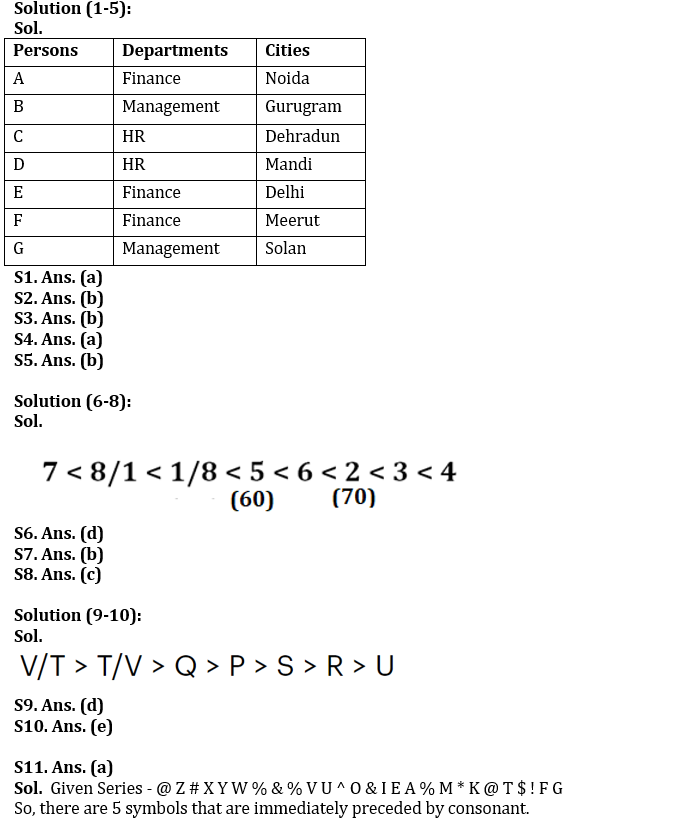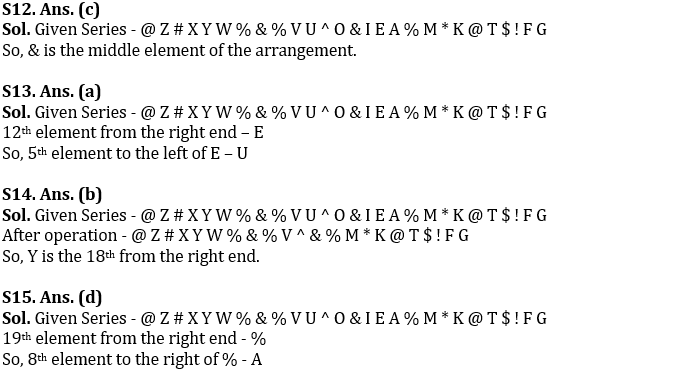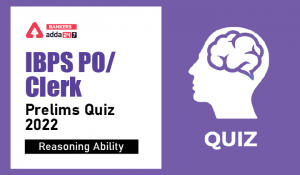Latest Banking jobs   »

# Reasoning Ability Quiz For IBPS RRB PO Clerk Prelims 2022- 13th July

Directions (1-5): Answer the questions based on the information given below.
Seven persons A, B, C, D, E, F, and G work in a company. They work in three different departments i.e., HR, Management and Finance. They belong to seven different cities such as Delhi, Dehradun, Meerut, Noida, Gurugram, Solan, and Mandi. At least two persons work in the same departments.
D belongs to Mandi and works in HR department. The one who belongs to Dehradun does not work in Finance department. G belongs to Solan and works in Management department with only B. A neither belongs to Gurugram nor works in HR department. E belongs to Delhi but does not work in HR department. F belongs to Meerut but does not work in HR department. No one from the HR department belongs to either Noida or Gurugram.

Q1. Who among the following works in the same department as C?
(a) Only D
(b) Only A and E
(c) Only F
(d) Only A
(e) None of the above

Q2. Who among the following doesn’t works in Finance department?
(a) A
(b) C
(c) E
(d) F
(e) All of the above

Q3. Who among the following belongs to Noida?
(a) C
(b) A
(c) Either A or C
(d) B
(e) Either A or B

Q4. C belongs to which of the following city?
(b) Meerut
(c) Gurugram
(d) Noida
(e) None of these

Q5. Which of the following is true regarding E?
(a) E – Gurugram – Management
(b) E – Delhi – Finance
(c) E – Dehradun – Finance
(d) E – Noida – Management
(e) None of the above

Directions (6-8): Answer the questions based on the information given below.
There are 8 storages (1, 2, 3, 4, 5, 6, 7 and 8) of Rice arranged in descending order of their weight from right to left. 5 is lighter than 6 but heavier than 8 and 1. 2 is heavier than 6. 7 is the lightest. 3 is heavier than 2 but not the heaviest. 4 is heavier than 6. The third heaviest weighs 70kg. There is a difference of 10kg between 5 and 2.

Q6. How many storages are heavier than 5?
(a) One
(b) Two
(c) Three
(d) Four
(e) None of the above

Q7. What is the possible weight of storage 6?
(a) 59kg
(b) 65kg
(c) 71kg
(d) 75kg
(e) None of the above

Q8. Which among the following is 2nd heaviest?
(a) 2
(b) 6
(c) 3
(d) 5
(e) None of the above

Directions (9-10): Answer the questions based on the information given below.
Seven persons P, Q, R, S, T, U, and V have different heights. Equal number of persons is taller and shorter than P. S is taller than R and U. Only V and T are taller than Q. R is not the shortest.

Q9. How many persons are taller than S?
(a) One
(b) Two
(c) Three
(d) Four
(e) None of the above

Q10. Who among the following is tallest?
(a) S
(b) R
(c) V
(d) T
(e) Cannot be determined

Directions (11-15): Study the following arrangement of symbols & alphabets carefully & answer the following questions:

@ Z # X Y W % & % V U ^ O & I E A % M * K @ T \$ ! F G

Q11. How many symbols are there in the arrangement which are immediately preceded by consonant?
(a) Five
(b) Eight
(c) Nine
(d) Ten
(e) None of the above

Q12. Which of the following is the middle element of the arrangement?
(a) ^
(b) O
(c) &
(d) I
(e) None of the above

Q13. Which of the following is the fifth element to the left of 12th element from right end?
(a) U
(b) V
(c) %
(d) O
(e) None of the above

Q14. If we drop all the vowels in the given arrangement then which of the following element is 18th from the right end?
(a) X
(b) Y
(c) %
(d) Z
(e) None of the above

Q15. Which of the following is the eighth element to the right of 19th element from right end?
(a) %
(b) M
(c) E
(d) A
(e) None of the above

Solutions#### Congratulations!Incorrect details? Fill the form again here

•Quantitative Aptitude Quiz For IBPS RRB ...
•Reasoning Ability Quiz For IBPS RRB PO C...
•Reasoning Ability Quiz For IBPS RRB PO C...
•Quantitative Aptitude Quiz For IBPS RRB ...
•Reasoning Ability Quiz For IBPS Clerk/PO...
•Reasoning Ability Quiz For IBPS RRB PO C...